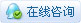##用户名 Email 自动登录 找回密码 密码 注册账号

《江湖公约》--行走江湖、必守规章 爆照有奖~还将成为我们的封面明星 侠客档案     申请会员     招募版主

• 江湖大转盘
• 参与人数:47 抽奖消耗:2金钱 每天可抽奖:1
 最新中奖布告
• 嘚嘚本人 抽中[谢谢参与]
• 人生如梦 抽中[谢谢参与]
• 惊无命 抽中[十等奖_论坛积分10]
• 嘚嘚本人 抽中[谢谢参与]
• 沉梦听雨 抽中[九等奖_子弹x2]
• 小知了 抽中[八等奖_绝情刀x3]
• 小九 抽中[六等奖_神牛令x5]
• 昨夜西风 抽中[六等奖_神牛令x5]
• 酒瓶子 抽中[谢谢参与]
• 莫问前程 抽中[十等奖_论坛积分10]
• 僵尸王 抽中[谢谢参与]
• 陌上清浅 抽中[谢谢参与]
• 以沫 抽中[谢谢参与]
• 惊无命 抽中[谢谢参与]
• 发热 抽中[九等奖_子弹x2]
• 江妄 抽中[六等奖_神牛令x5]
• 僵尸王 抽中[谢谢参与]
• 梦飞雪。。 抽中[谢谢参与]
• 小九 抽中[谢谢参与]
• 白发浴红衣 抽中[三等奖_攻防卡]
• 永离尘情 抽中[八等奖_绝情刀x3]
• 人生如梦 抽中[谢谢参与]
• 肥尸 抽中[谢谢参与]
• 池小晚 抽中[十等奖_论坛积分10]
• 昨夜西风 抽中[谢谢参与]
• 陌上清浅 抽中[谢谢参与]
• 半世琉璃 抽中[七等奖_水晶球x4]
• 嘚嘚本人 抽中[八等奖_绝情刀x3]
• 莫问前程 抽中[谢谢参与]
• 酒瓶子 抽中[五等奖_灵葫仙丹卡]
• 沉梦听雨 抽中[十等奖_论坛积分10]
• 飒雪飞歌 抽中[十等奖_论坛积分10]
• 香儿 抽中[谢谢参与]
• 陌上清浅 抽中[八等奖_绝情刀x3]
• 粉红淘桃 抽中[五等奖_灵葫仙丹卡]
• 嘚嘚本人 抽中[六等奖_神牛令x5]
• 僵尸王 抽中[谢谢参与]
• 五月糖 抽中[谢谢参与]
• 一生潇潇 抽中[十等奖_论坛积分10]
• 人生如梦 抽中[四等奖_天仙玉露卡]
• 雪蝶 抽中[十等奖_论坛积分10]
• 肥尸 抽中[九等奖_子弹x2]
• 小乖小松子 抽中[八等奖_绝情刀x3]
• 风吹牛儿走 抽中[九等奖_子弹x2]
• 多多 抽中[六等奖_神牛令x5]
• 李斯特 抽中[十等奖_论坛积分10]
• 小九 抽中[谢谢参与]
• 酒瓶子 抽中[十等奖_论坛积分10]
• 池小晚 抽中[谢谢参与]
• 半世琉璃 抽中[谢谢参与]
• 莫问前程 抽中[八等奖_绝情刀x3]
• 烈如歌 抽中[八等奖_绝情刀x3]
• 沉梦听雨 抽中[九等奖_子弹x2]
• 安夏 抽中[谢谢参与]
• 白发浴红衣 抽中[六等奖_神牛令x5]
• 永离尘情 抽中[十等奖_论坛积分10]
• 飒雪飞歌 抽中[谢谢参与]
• 惊无命 抽中[谢谢参与]
• 杳言 抽中[谢谢参与]
• 雪落无痕 抽中[谢谢参与]
• 嘚嘚本人 抽中[五等奖_灵葫仙丹卡]
• 由我不由天 抽中[十等奖_论坛积分10]
• 肥尸 抽中[九等奖_子弹x2]
• 雪蝶 抽中[十等奖_论坛积分10]
• 寂瞳 抽中[十等奖_论坛积分10]
• 梦飞雪。。 抽中[七等奖_水晶球x4]
• 人生如梦 抽中[谢谢参与]
• 五月糖 抽中[十等奖_论坛积分10]
• 小乖小松子 抽中[谢谢参与]
• 酒瓶子 抽中[谢谢参与]
• 沉梦听雨 抽中[谢谢参与]
• 池小晚 抽中[十等奖_论坛积分10]
• 半世琉璃 抽中[谢谢参与]
• 陌上清浅 抽中[谢谢参与]
• 小九 抽中[六等奖_神牛令x5]
• 烈如歌 抽中[十等奖_论坛积分10]
• 静候轮回 抽中[谢谢参与]
• 李斯特 抽中[谢谢参与]
• 风行水止 抽中[十等奖_论坛积分10]
• 僵尸王 抽中[谢谢参与]
• 风吹牛儿走 抽中[谢谢参与]
• 小知了 抽中[十等奖_论坛积分10]
• 以沫 抽中[八等奖_绝情刀x3]
• 嘚嘚本人 抽中[谢谢参与]
• 风吹牛儿走 抽中[谢谢参与]
• 山里朵 抽中[谢谢参与]
• 龍戈兒 抽中[谢谢参与]
• 神书飞扬 抽中[十等奖_论坛积分10]
• 安夏 抽中[六等奖_神牛令x5]
• 风行水止 抽中[十等奖_论坛积分10]
• 惊无命 抽中[谢谢参与]
• 陌上清浅 抽中[谢谢参与]
• 小知了 抽中[谢谢参与]
• 行龙不雨 抽中[谢谢参与]
• 风雨飘摇 抽中[十等奖_论坛积分10]
• 陌桑。 抽中[十等奖_论坛积分10]
• 人生如梦 抽中[八等奖_绝情刀x3]
• 烈如歌 抽中[谢谢参与]
• 江妄 抽中[谢谢参与]
• 小九 抽中[谢谢参与]
• 发热 抽中[谢谢参与]
• 飒雪飞歌 抽中[七等奖_水晶球x4]
• 酒瓶子 抽中[六等奖_神牛令x5]
• 多多 抽中[谢谢参与]
• 池小晚 抽中[六等奖_神牛令x5]
• 半世琉璃 抽中[谢谢参与]
• 以沫 抽中[谢谢参与]
• 香儿 抽中[谢谢参与]
• 小乖小松子 抽中[八等奖_绝情刀x3]
• 色心寻胆 抽中[十等奖_论坛积分10]|手机版|Archiver|小黑屋|醉江湖论坛 |网站地图